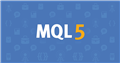# Improving Speed of Code Execution

Hi everyone
I am writing an MT5 multi-currency  EA that uses several indicators.

Rather than create Handles and Arrays for each Indicator/Currency I created functions to easily get the values for each indicator  that I use in the OnTick on every new candle.
It takes however rather long to calculate.
Can anyone suggest an alternative?
Thank you

The functions

```//+------------------------------------------------------------------+
//|                                                                  |
//+------------------------------------------------------------------+
double Ma_Function(
string               symbol,            // symbol name
ENUM_TIMEFRAMES      period,            // period
int                  ma_period,         // averaging period
int                  ma_shift,          // horizontal shift
ENUM_MA_METHOD       ma_method,         // smoothing type
ENUM_APPLIED_PRICE   applied_price, // applied price
int                  buffernumber=0,   //buffer number
int                  shift=0   //shift

)
{

double valueArray[];
ArraySetAsSeries(valueArray,true);
int Handle=iMA(symbol,period,ma_period,ma_shift,ma_method,applied_price);
CopyBuffer(Handle,buffernumber,shift,1,valueArray);

double value=valueArray;
return value;

}

//+------------------------------------------------------------------+
//|                                                                  |
//+------------------------------------------------------------------+
double MACD_Function(
string              symbol,              // symbol name
ENUM_TIMEFRAMES     period,              // period
int                 fast_ema_period,     // period for Fast average calculation
int                 slow_ema_period,     // period for Slow average calculation
int                 signal_period,       // period for their difference averaging
ENUM_APPLIED_PRICE  applied_price,        // type of price or handle
int                  buffernumber=0,   //buffer number
int                  shift=0   //shift
)
{

double valueArray[];
ArraySetAsSeries(valueArray,true);

int Handle=iMACD(symbol,period,fast_ema_period, slow_ema_period,signal_period,applied_price);
CopyBuffer(Handle,buffernumber,shift,1,valueArray);

double value=valueArray;
return value;
}
//+------------------------------------------------------------------+
//|                                                                  |
//+------------------------------------------------------------------+
double RSI_Function(
string              symbol,              // symbol name
ENUM_TIMEFRAMES     period,              // period
int                 ma_period,     // period for Fast average calculation
ENUM_APPLIED_PRICE  applied_price,        // type of price or handle
int                  buffernumber=0,   //buffer number
int                  shift=0   //shift
)
{

double valueArray[];
ArraySetAsSeries(valueArray,true);
int Handle=iRSI(symbol,period,ma_period,applied_price);
CopyBuffer(Handle,buffernumber,shift,1,valueArray);

double value=valueArray;
return value;
}```

And I use the functions in the OnTick of the code on every new bar to get the values

```for (int i=0; i<ArraySize(CurrencyList); i++)
{
symbol=CurrencyList[i];
double maLong=Ma_Function(symbol,periodos,MaLongLength,0,MaLongMethod,PRICE_CLOSE,0,shift);
double maMed=Ma_Function(symbol,periodos,MaMedLength,0,MaMedMethod,PRICE_CLOSE,0,shift);
double maShrt=Ma_Function(symbol,periodos,MaShrtLength,0,MaShrtMethod,PRICE_CLOSE,0,shift);
double macd= MACD_Function(symbol,periodos,MACDFastEMA,MACDSlowEMA,MACDSMA,PRICE_CLOSE,0,shift);
double rsi=RSI_Function(symbol,periodos,RSItrendlength,PRICE_CLOSE,0,shift);

}
```Documentation on MQL5: Constants, Enumerations and Structures / Indicator Constants / Indicator Types
• www.mql5.com
There are two ways to create an indicator handle for further accessing to its values. The first way is to directly specify a function name from the list of technical indicators. The second method using the IndicatorCreate() is to uniformly create a handle of any indicator by assigning an identifier from the ENUM_INDICATOR...
Reason: